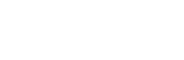#0.0000 0 3 关注作者 收藏

## 评论(1)2019-02-20

#I called mine Ancovas. --> Note, export your df as .csv to work with it in R.

#Next, subset your data by the two conditions (e.g. "l"=light, "d"=dark), and both treatments (e.g. "MQ"=water, "DOM"=media)

AncovasL <- Ancovas[(Ancovas\$UV == "Light"), ]

AncovasL.MQ <- AncovasL[(AncovasL\$DOM == "MQ"), ]

AncovasL.DOM <- AncovasL[(AncovasL\$DOM == "DOM"), ]

AncovasD <- Ancovas[(Ancovas\$UV == "Dark"), ]

AncovasD.MQ <- AncovasD[(AncovasD\$DOM == "MQ"), ] #This code only keeps what is inside the brackets

AncovasD.DOM <- AncovasD[(AncovasD\$DOM == "DOM"), ] #Note, adding and "!" after square bracket removes what is in " ".

#--> this code was gathered from several sites.

#note: I don't understand the logic of how the numbers in brackets are organized. But this essentially pulls some information from the fit model. i.e.  means find the 9th value in the list (I think)

regression = function(Ancovas){

fit <- lm(AvgBio ~ Exposure, data=Ancovas)

slope <- round(coef(fit),1)

intercept <- round(coef(fit),0)

R2 <- round(as.numeric(summary(fit)),3)

p.val <- signif(summary(fit)\$coef[2,4], 3)

regressions_dataL.MQ <- ddply(AncovasL.MQ, "Treatment", regression) #For light samples using water

regressions_dataL.DOM <- ddply(AncovasL.DOM, "Treatment", regression) #For light samples using media

regressions_dataD.MQ <- ddply(AncovasD.MQ, "Treatment", regression) #For dark samples using water

regressions_dataD.DOM <- ddply(AncovasD.DOM, "Treatment", regression) #For dark samples using media

#Rename columns

#Yes I like to hyper control every aspect of my theme

theme_new <- theme(panel.background = element_rect(fill = "white", linetype = "solid", colour = "black"),

legend.key = element_rect(fill = "white"), panel.grid.minor = element_blank(), panel.grid.major = element_blank(),

axis.text.x=element_text(size = 11, angle = 0, hjust=0.5), #axis numbers (set it to 1 to place it on left side, 0.5 for middle and 0 for right side)

axis.text.y=element_text(size = 13, angle = 0),

plot.title=element_text(size=15, vjust=0, hjust=0), #hjust 0.5 to center title

axis.title.x=element_text(size=14), #X-axis title

axis.title.y=element_text(size=14, vjust=1.5), #Y-axis title

legend.position = "top",

legend.title = element_text(size = 11, colour = "black"), #Legend title

legend.text = element_text(size = 8, colour = "black", angle = 0), #Legend text

strip.text.x = element_text(size = 9, colour = "black", angle = 0), #Facet x text size

strip.text.y = element_text(size = 9, colour = "black", angle = 270)) #Facet y text size

guides_new <- guides(color = guide_legend(reverse=F), fill = guide_legend(reverse=F)) #Controls the order of your legend

Colours <-

rainbow_hcl(length(levels(factor(StackedTable\$DOM))), start = 30, end = 300) #Yes I am Canadian so Colours has a "u"

Colours <- "#47984c" #Green

Colours <- "#7b64b4" #Purple-grey

Colours <- "#ff7f50" #Orange

Colours <- "#cc3636" #Red

Colours <- "#4783ba" #Blue

#Making plot for panel A ("Dark condition")

PlotA <-

ggplot(AncovasD, aes(x=as.numeric(Time.h), y=as.numeric(Measurement), fill=as.factor(Treatment))) +

geom_smooth(data=subset(AncovasD,Treatment =="MQ"), aes(Time.h,Measurement,color=factor(Treatment)),method="lm", formula = y~x, se=T, show.legend = F) +

geom_smooth(data=subset(AncovasD,Treatment =="DOM"), aes(Time.h,Measurement,color=factor(Treatment)),method="lm", formula = y~x, se=T, show.legend = F) + #You need this line twice, once for each condition

geom_errorbar(data=AncovasD, aes(ymin=Measurement-SD, ymax=Measurement+SD), width=0.2, colour="#73777a", size = 0.5) + #Change width based on the size of your X-axis

geom_point(shape = 21, size = 3, colour = "black", stroke = 1) + #colour is the outline of the circle, stroke is the thickness of that outline

facet_grid(Treatment ~ UV) + #This places all your treatments into a grid. Change the order if you want them horizontal. Use "." if you do not want a label.

geom_label(data=regressions_dataD.MQ, inherit.aes=FALSE, size=0.7, colour=Colours, #Add label for DOM regressions, specify same colour as your legend, change size depending on how large you want the text

aes(x=-0.1, y=41, label=paste(" ", "m == ", slope, "\n " , #replace this line with the values you want: e.g. R-squared=("R2 == ", R2.Adj) ; intercept=("b == ", intercept). The "\n " makes a second line

" ", "p == ", p.val ))) + #This completes the first label. Repeat same process for second label.

aes(x=-0.1, y=4, label=paste(" ", "m == ", slope, "\n " ,

" ", "p == ", p.val )))

#Now for the irradiated samples "light" plot (Panel B)

PlotB <-

ggplot(AncovasL, aes(x=as.numeric(Time.h), y=as.numeric(Measurement), fill=as.factor(Treatment))) + #Same as above but use your second dataframe.

geom_smooth(data=subset(AncovasL,Treatment =="MQ"), aes(Time.h,Measurement,color=factor(Treatment)),method="lm", formula = y~x, se=T, show.legend = F) +

geom_smooth(data=subset(AncovasL,Treatment =="DOM"), aes(Time.h,Measurement,color=factor(Treatment)),method="lm", formula = y~x, se=T, show.legend = F) +

geom_errorbar(data=AncovasL, aes(ymin=Measurement-SD, ymax=Measurement+SD), width=0.2, colour="#73777a", size = 0.5) +

geom_point(shape = 21, size = 3, colour = "black", stroke = 1) +

facet_grid(Treatment ~ UV) +

geom_label(data=regressions_dataL.MQ, inherit.aes=FALSE, size=0.7, colour=Colours,

aes(x=-0.1, y=41, label=paste(" ", "m == ", slope, "\n " ,

" ", "p == ", p.val ))) +

geom_label(data=regressions_dataL.DOM, inherit.aes=FALSE, size=0.7, colour=Colours,

aes(x=-0.1, y=4, label=paste(" ", "m == ", slope, "\n " ,

" ", "p == ", p.val )))

0.0000 0 0 回复